# Use The Onion Method To Approach Complex Formulas

Complex Formulas? The Onion Method? Huh?

I’m talking about the idea that complex formulas in Google Sheets are a lot like onions.

They have layers.

And they sometimes make you cry. 🤣

## The Onion Method For Complex Formulas

If you’re building complex formulas, then I advocate following a one-action-per-step approach.

What I mean by this is that you build your formulas in a series of steps, and only make one change with each step.

The Onion Method is a framework by which to approach hard formulas, and consists of these three elements:

1. Put each new step of the formula in a new cell
2. Label each step with a simple “Step 1”, “Step 2”, etc. in adjacent cells
3. Change the background color of each formula cell, so they can be easily found

This lets you see the formula progress in an incremental way and is really helpful when you’re building or tyring to understand complex formulas.

Sometimes a step might result in an error (typically a #N/A or #REF!), but that’s ok, provided it gets fixed in a subsequent step, as shown in this SUMPRODUCT example: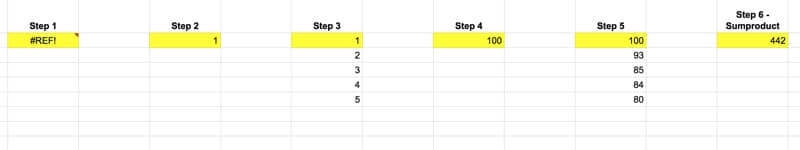Each of these intermediary formulas in the above image moves us forward incrementally, until the final answer is obtained in step 6.

Similarly, if you’re trying to understand complex formulas, peel the layers back until you reach the core (which is hopefully a function you understand!). Then, build it back up in steps to get back to the full formula.

## Example 1: Building A Complex Formula With The Onion Method

Let’s look at importing data from the table on this Wikipedia page of largest cities by population.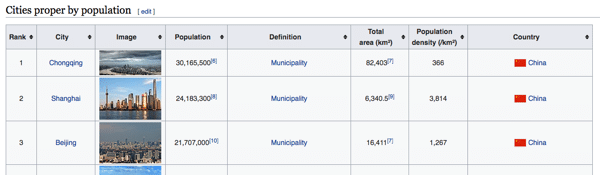### Step 0

Open a new Google Sheet (bonus points for using the browser shortcut and just typing `Sheets.new` into your browser window).

### Step 1

Step 1 is a standard IMPORTHTML function to retrieve the city table from Wikipedia. In cell A2:

`=IMPORTHTML("https://en.wikipedia.org/wiki/List_of_cities_proper_by_population","table",2)`

The data has some issues, but it’s a start.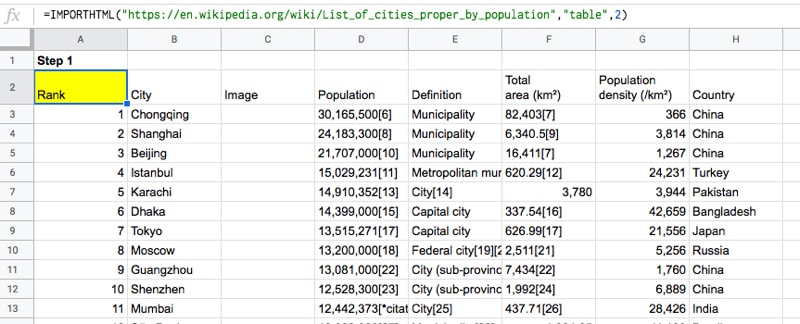### Step 2

In Step 2, we use the INDEX function to grab just the population column.

Per The Onion Method, we insert this next step in a new cell, cell J2, to the right of the existing data, in this example:

`=INDEX(IMPORTHTML("https://en.wikipedia.org/wiki/List_of_cities_proper_by_population","table",2),,4)`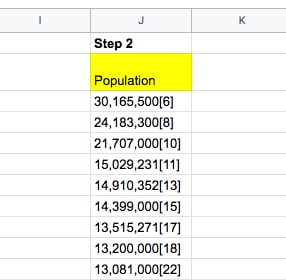I’ve highlighted the new action step in red — adding the INDEX function wrapper.

Looking closely at that INDEX function wrapper, you’ll see that we’ve left the row argument blank, namely:

`INDEX(data, ,4)`

which returns the entire column.

We’ll deal with the population column on its own and come back to our main formula later.

### Step 3

Hmm, that population column is messed up! Regex to the rescue!

Using the REGEXEXTRACT function, extract just the numbers and “,” from the data, before the citations in brackets:

`=REGEXEXTRACT(INDEX(IMPORTHTML("https://en.wikipedia.org/wiki/List_of_cities_proper_by_population","table",2),,4),"[0-9,]+")`

Hmm, that gives us a #N/A error… 🤔

### Step 4

Turn this into an Array Formula and get the column of population numbers!

`=ArrayFormula(REGEXEXTRACT(INDEX(IMPORTHTML("https://en.wikipedia.org/wiki/List_of_cities_proper_by_population","table",2),,4),"[0-9,]+"))`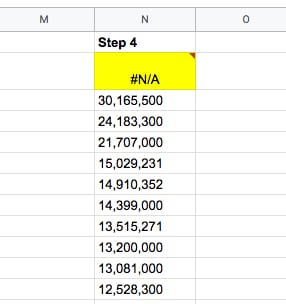We still have two problems to solve though: i) we need to convert the strings into actual numbers and ii) we need to fix the #N/A column heading…

### Step 5

We can multiply by 1 (see the “*1” at the end of our formula), which coerces those text strings into actual numbers.

`=ArrayFormula(REGEXEXTRACT(INDEX(IMPORTHTML("https://en.wikipedia.org/wiki/List_of_cities_proper_by_population","table",2),,4),"[0-9,]+") * 1)`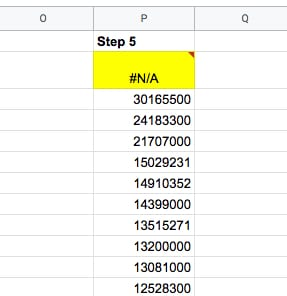### Step 6

Use the IFERROR function to fix that pesky #N/A error at the top of our column heading, and replace the #N/A with the word “Population”:

`=ArrayFormula(IFERROR(REGEXEXTRACT(INDEX(IMPORTHTML("https://en.wikipedia.org/wiki/List_of_cities_proper_by_population","table",2),,4),"[0-9,]+")*1,"Population"))`

Nice, now we have our population column as numbers:### Step 7

Pick the other columns we want, by wrapping the IMPORTHTML function with a QUERY function.

Note that we have to use the Col1 notation rather than the column letter in our Select statement, since we’re nesting another function as our data source in the QUERY function.

We also use the QUERY function rather than the INDEX function because we want to return multiple columns this time, which the INDEX function can’t do.

`=QUERY(IMPORTHTML("https://en.wikipedia.org/wiki/List_of_cities_proper_by_population","table",2),"SELECT Col2, Col8",1)`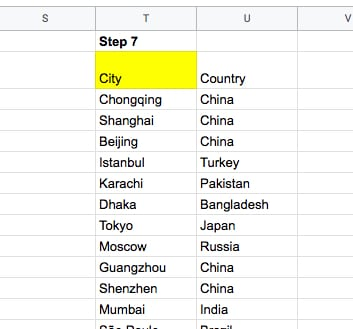### Step 8

All that’s left is to join these two ranges using the curly bracket notation, like this:

```={ QUERY(IMPORTHTML("https://en.wikipedia.org/wiki/List_of_cities_proper_by_population","table",2),"SELECT Col2,Col8",1) , ArrayFormula(IFERROR(REGEXEXTRACT(INDEX(IMPORTHTML("https://en.wikipedia.org/wiki/List_of_cities_proper_by_population","table",2),,4),"[0-9,]+") * 1,"Population")) ```

`}`

(Shown with line breaks to illustrate the two ranges.)

Ok, we’re done 🤪

The output is:## Example 2: Deconstructing With The Onion Method

If you’re trying to understand complex formulas in Google Sheets that someone else has shared with you, you can still approach it with this Onion Method.

Simply peel back the layers until you reach the innermost function. Copy that into a new cell and start from the inside and work out, building up to the full formula again.

Let’s see an example.

Suppose we’re given this worksheet with US State names: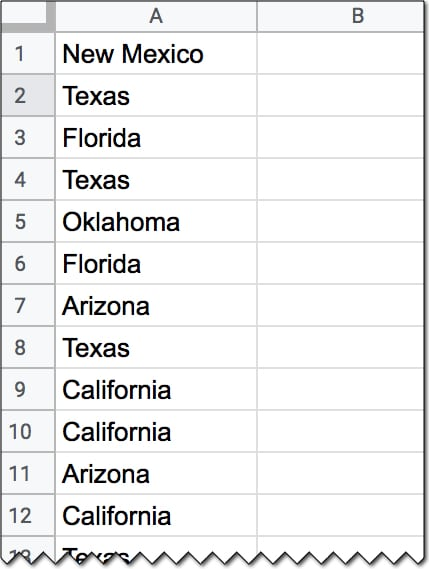And we’re also given this formula:

`=ArrayFormula(INDEX(A1:A20,MODE(MATCH(A1:A20,A1:A20,0))))`

which gives an output of Texas.

But how does this formula work?

Applying The Onion Method, we peel back the layers to the core function, and then build it up in steps again.

### Step 1

In a new cell, add the innermost MATCH function:

`=MATCH(A1:A20,A1:A20,0)`

### Step 2

`=ArrayFormula(MATCH(A1:A20,A1:A20,0))`

which outputs an array of the position of the first occurrence of the words in column A. We see a 2 next to every occurrence of Texas for example, because the first time it occurred was in position 2.

### Step 3

Now, we wrap it with the MODE function to find the most frequently occurring position:

`=ArrayFormula(MODE(MATCH(A1:A20,A1:A20,0)))`

By definition, the MODE function takes a range of numbers for an input and finds the most commonly occurring value.

However, what happens if we have a range of text values and want to find the most frequent?

In this case, the MATCH has been used to create a range of numbers for the MODE function.

By now, we’ve probably deduced that this formula finds the most frequent word in a list.

### Step 4

Finally, we can retrieve the actual text value, i.e. the most frequent State name, by adding the INDEX function to get the full original formula, like this:

`=ArrayFormula(INDEX(A1:A20,MODE(MATCH(A1:A20,A1:A20,0))))`

This will give the output Texas in this specific example.

Nice!

This template contains both examples from this tutorial.

To make your own editable copy, please go to File > Make a copy… under the File menu.

## Conclusion

The Onion Method is a framework that allows you to approach complex formulas in a systematic way.

Even if you’re presented with an “impossible” challenge to answer or an “impossible” formula to decipher, just follow this framework. If required, peel back the layers and then work from the inside out in an incremental fashion.

You’ll be amazed at how quickly your understanding of challenging formulas broadens and deepens. You’ll encounter and understand brand new functions that you’ve never heard of before. Plus, you’ll find out all sorts of secret tricks with existing formulas.

Who knows, you might even cry tears of joy instead of despair…

I’ll leave you with this quote from businesswoman Belinda Johnson:

I like cutting through complexity and trying to get to the kernel of an idea.

## 20 thoughts on “Use The Onion Method To Approach Complex Formulas”

1.Dave says:

Excellent post on complex formulas. What are your thoughts on using an outside program in the Google suite to put together epic long formulas in Sheets? I’ve tried this with limited success in Docs since some of the formatting for the formula appears to be lost copying and pasting from Docs to Sheets.

1.Ben says:

Hey Dave,

Yes, I think it can be helpful! I actually use Sublime Text myself (a fancy text editor for coding) to write out really long formulas. It’s helpful to split them over multiple lines, indent etc. The formatting ports across no problem, but I know copying and pasting formulas from the web can sometimes be problematic, e.g. with quotation signs messing up.

Cheers,
Ben

2.Joel says:

Thanks Ben

Something I found really helped me get through very complex layered formulas is to essentially build them layer by layer IN the actual formula bar with 1 line per layer rule (ish!).
The formula bar itself can be pulled down to make it a bigger working window and then you can write each later independently
e.g. I have a formula that looks for names in an agent table and then repeats them 10 times before positioning the next name…

So where the actual formula is this:
))) & “\|”, 10), “|”))), “\”)))

I built it and READ it this way, which makes it far easier to find and replace any issues/errors or to edit it for other uses or updates.
= sort
(transpose(
split(
concatenate(
arrayformula(
split(
rept(
unique(
(filter
)))
& “\|”, 10),
“|”)))
, “\”)))

1.Ben says:

Thanks for sharing, Joel!

I agree that writing formulas over multiple lines can be really helpful when they get really long. Something I’ve found recently though is that the line breaks are not persisted by Google. When you exit the formula and come back, it’s reverted to the formula all being squashed up again. Haven’t figured out if there’s a way to avoid this yet…

Cheers,
Ben

1.Gary says:

This is strange behaviour. I tried it in one of my Sheets. =IMPORTRANGE(SheetLastMonth,”TDC!H5:J8″) in one cell remains on one line no matter what, but elsewhere on the same tab I have no trouble getting =IMPORTRANGE(
SheetLastYear,”TDC!F14:F29″) to persist over two lines.

Scratch that. I reopened the file and found the formula on one line is now on two and deleting the newline doesn’t work; it stays on two lines. Bizarre, and too random to rely on 🙁

1.Ben says:

Yes! I agree. I’ve found that it works sometimes, but often not. If I write a formula in an external editor, with line breaks, and copy that into the formula bar then I find they persist and stay in place then.

2.DeeKay789 says:

…. make an irrelevant/obvious change. Press enter. Go back and it’s formatted as you left it. Re-instate the obvious change. Press enter. Go back in and it’s all still formatted as you left it. I think it’s a Google cache optimisation. FWIW. Thx for all you insights Ben.
D

3.JOEL MCLEAN says:

I’ve found using spaces (extra spaces wont impact the actual formula) after each line break helps remove this issue.

3.Davo says:

Using a more systemic approach like this also makes it easier for other people to maintain documentation as it’s easier to understand what the creator of the formula had in mind by following the different steps.

This is important to keep in mind when we create spreadsheets that ware expected to work for our teams whether we are part of the team/project or not.

1.Ben says:

Totally! That’s a great point, Davo. Thanks for sharing.

4.Reed says:

Thanks for the post, excellent information. I am a big fan of the IFERROR to clean up data and provide a structure to my tables.

1.Ben says:

You’re welcome! IFERROR is a very handy function.

5.JhowTro Mundo says:

Great.
I laughed or cried, complex formulas I wrote

1.Ben says:

Or both at the same time 😂

6.Anne says:

Thank you very much Ben, for these great examples.
I often use the onion construction when I have to use more than 2 functions because for some reason, it never works properly the first time. I found it easier to understand how formulas work, and go easier to the result.
Thank you for sharing so much!

1.Ben says:

You’re welcome! It definitely makes things easier to understand 😉

7.Keith says:

Great process and great timing. I recently spent a bit creating a custom function in apps script. Super easy to read and maintain. I was pretty happy with it. Only to find that it constantly errors with

“Error
Service invoked too many times in a short time: exec qps. Try Utilities.sleep(1000) between calls. (line 0).”

So now I get to recreate it with built in functions, outside of the script editor. This process will help. Thanks!

8.Octave says:

Here a challenge I can not resolve …and maybe you could explain to me how resolve it ..with The Onion Method For Complex Formulas

this is like a special transpose data
here is my public url spreadsheet

THANKS in advance to everyone could help me or could give me a link to solve it.

9.Matt Taylor says:

Ben, the formatting of the source data has changed.
Instead of suffixing the population with the contents of the square brackets, it is now prefixing it. As such the regex no longer works as is.

I believe I was able to resolve this (after many, much more complicated attempts, having overlooked the simply solution) by changing the regex from
[0-9,]+
to
[0-9,]+\$

Thanks for the website by the way. Really helping with my new job!